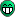# Fitting a histogram with a poisson function

Hi there,

I’m trying to fit a histogram (‘value’) with a poisson function.
The following code is what I tried:

`````` f1 = ROOT.TF1("f1","*ROOT.TMath.Power((/),(x/))*(ROOT.TMath.Exp( (/)))/ROOT.TMath.Gamma((x/)+1)", 0, 5)
f1.SetParameters(1, 1, 1)
values.Fit('f1', 'R')
fit = values.GetFunction('f1')
fit.Draw('same')``````

However I get the following error and I don’t know why:

``````Error in <TFormula::Compile>:  Bad numerical expression : "ROOT.TMath.Power((/),(x/))"
Error in <TF1::TF1>: function: f1/*ROOT.TMath.Power((/),(x/))*(ROOT.TMath.Exp(-(/)))/ROOT.TMath.Gamma((x/)+1) has 0 parameters instead of 1
Unknown function: f1
Traceback (most recent call last):
File "Ansprechmuster3.py", line 242, in <module>
fit.Draw('same')
ReferenceError: attempt to access a null-pointer
``````

So something is wrong with “`ROOT.TMath.Power((/),(x/))`” but I really don’t have an idea.
Does anybody know what’s the problem?

Try:

``````f1 = ROOT.TF1("f1", "*TMath::Power((/),(x/))*(TMath::Exp( (/)))/TMath::Gamma((x/)+1)", 0, 5)
``````

Thanks for the suggestion but while I was waiting for an answer I tried this and it worked too:

``````def fitfunction_poisson(x, par):
if par != 0:
if ROOT.TMath.Gamma((x/par)+1) != 0:
poisson = par * ROOT.TMath.Power((par/par),(x/par)) * (ROOT.TMath.Exp(-(par/par)))/ROOT.TMath.Gamma((x/par)+1)
else:
poisson = 0
else:
poisson = 0

return poisson
PoissonFit = ROOT.TF1('PoissonFit', fitfunction_poisson, 0, 5, 3)
PoissonFit.SetParameter(0, 1)
PoissonFit.SetParameter(1, 1)
PoissonFit.SetParameter(2, 1)
PoissonFit.SetParNames('par0', 'par1', 'par2')
PoissonFit.SetLineColor(4)
PoissonFit.SetLineWidth(2)
PoissonFit.SetLineStyle(2)

ROOT.gStyle.SetOptFit(0111)

values.Fit('PoissonFit', 'R')
PoissonFit.Draw('same')``````

However I’ve got a new problem now:
Despite setting SetOptFit to 0111 (like it is said in the ROOT manual) the reduced chi square isn’t shown in the statistics box (parameters 0-2 are displayed though).
Any idea why?Try: `gStyle->SetOptFit(0157);`For some additional hints see: Only display errors and name/values of parameters in a fitWorked, thank you very much!

However I have to say that the ROOT manual is very misleading because there’s no mention of any octals and it clearly says that 0111 should do the job